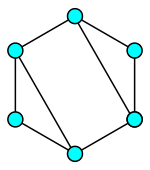## Graph - Weekly Travelling in CPAN

Destination: Graph
Date of Latest Release: Feb 12, 2023
Distribution: Graph
Module version: 0.9726
Main Contributors: Jarkko Hietaniemi (JHI)
Current Maintainer: Neil Bowers (NEILB)Long time ago I claimed in front of a friend I would write a short introduction to graph theory, but I had been not able to figure out where I should start. Neither I would try today. The mathematical objects graphs, or the abstract data structures graphs, are full of interesting behaviors being studied in the discrete math subdiscipline graph theory. The CPAN module Graph is designed to empower Perl programmers doodle with undirected graphs and directed graphs (and also multigraphs and hypergraphs - not going to visit these functionalities here).

```use v5.30.0;
use warnings;
use Graph::Undirected;

my \$g = Graph::Undirected->new;

for my \$x (2..19) {
for my \$y (\$x+1..20) {
\$g->add_edge(\$x, \$y) if !(\$x % \$y) || !(\$y % \$x);
}
}

my @cc = \$g->connected_components;

my \$mst = \$g->MST_Kruskal;

use Data::Printer;
p @cc;
p \$mst; ```

A graph is basically consist of its vertices and edges. In the Graph module, you can use scalar values or variables as vertices. In the above code snipplet, the graph vertices are "labelled" with integers from 2 to 20, then two vertices are connected by an edge if a number is divisible by another number.

Result:
```[
 [
  18,
  6,
  3,
...
 14,
 7
],
 [
 13
],
 [
 19
],
 [
 17
],
 [
 11
]
]
10=2,10=5,12=2,14=2,14=7,15=3,16=2,18=2,2=20,2=4,2=6,2=8,3=6,3=9 (Graph)```

Let see an example of graph vertices labelling with variables. Suppose the data structure [ \$name0, [@array_of_names] ] represents the person of \$name0 have friends with names in @array_of_names.

```use v5.30.0;
use warnings;
use Graph::Undirected;

my \$alice = ["Alice", ["Bob"]];
my \$bob   = ["Bob", ["Alice", "Cathy", "David"]];
my \$david = ["David", ["Bob"]];

my \$g = Graph::Undirected->new;

for my \$x (\$g->vertices) {
for my \$y (\$g->vertices) {
\$g->add_edge(\$x, \$y) if   grep(\$_ eq \$y->, \$x->->@*)
&& grep(\$_ eq \$x->, \$y->->@*);
}
}

my @cc = \$g->connected_components;

say scalar @cc; #1   These 3 people are in a community.```

We may also express our idea in the last line as: say "Alice, Bob and David are in a community" if \$g->is_connected;

In an ideal world, your friends treat you as a friend. Let us face a non-ideal world.

```use v5.30.0;
use Graph::Directed;
use Graph::Undirected;
use warnings;

my \$alice = ["Alice", ["Bob"]];
my \$bob   = ["Bob", ["Alice", "Cathy"]];
# today Bob is no longer friendly towards David
my \$cathy = ["Cathy", []];
my \$david = ["David", ["Bob"]];

my \$g = Graph::Directed->new;

for my \$x (\$g->vertices) {
for my \$y (\$g->vertices) {
\$g->add_edge(\$x, \$y) if grep(\$_ eq \$y->, \$x->->@*);
}
}

my \$gu = Graph::Undirected->new;
for my \$x (\$gu->vertices) {
for my \$y (\$gu->vertices) {
\$gu->add_edge(\$x, \$y) if \$g->has_edge(\$x, \$y) && \$g->has_edge(\$y, \$x);
}
}

say "People live in a connected world" if \$g->undirected_copy->is_connected;
# printed
say "People are in a community" if \$gu->is_connected;
# not printed :(```

Finally is my recent use case of the module. It is from The Weekly Challenge 209 Task 2. You can read the problem statement here.

The main strategy in the following code is treating each vertex as an email address, connecting two email addresses if they are belonged to the same account. Then find out each connected component of the graph is an account after merging. (I modified the name of the account, originally it is only "A" and "B"; I think the essence of the scenario is more obvious.)

```use v5.30.0;
use warnings;
use Graph::Undirected;

my @accounts1 = (    ['Alex', 'a1@a.com', 'a2@a.com'],
['Bob', 'b1@b.com'],
['Alexender', 'a3@a.com', 'a1@a.com'] );

my @accounts2 = (    ['Alex Smith', 'a1@a.com', 'a2@a.com'],
['Bob', 'b1@b.com'],
['Alex Wicks', 'a3@a.com'],
['Bobby', 'b2@b.com', 'b1@b.com'] );

sub merge_acc {
my @accs = @_;
my %email;
for my \$acc (@accs) {
for my \$i (1..\$acc->\$#*) {
\$email{\$acc->[\$i]} = \$acc->;
}
}
my \$g = Graph::Undirected->new;
for my \$acc (@accs) {
for my \$i (1..\$acc->\$#*-1) {
# can be enhanced to add edges between more emails
}
}
my @cc = \$g->connected_components;
my @ans = ();
for my \$c (@cc) {
unshift \$c->@*, \$email{\$c->};
push @ans, \$c;
}
return @ans;
}

my @result1 = merge_acc(@accounts1);
my @result2 = merge_acc(@accounts2);

=pod
use Data::Printer;
p @result1;
p @result2;

# The account name chosen and the order
# of the email addresses is not
# the same each time.

[
 [
 "Alexender",
 "a1@a.com",
 "a2@a.com",
 "a3@a.com"
],
 [
 "Bob",
 "b1@b.com"
]
]
[
 [
 "Bobby",
 "b2@b.com",
 "b1@b.com"
],
 [
 "Alex Smith",
 "a1@a.com",
 "a2@a.com"
],
 [
 "Alex Wicks",
 "a3@a.com"
]
]
```

People who want to study the introduction of graph theory can read the following free resources:
https://www.whitman.edu/mathematics/cgt_online/book/

And there is a nice drawing tool from the Elm language community:
https://erkal.github.io/kite/

THE HIGHLIGHTED PERL MODULES OF WEEK 13 OF 2023:
GraphThis blog is inactive and replaced by https://e7-87-83.github.io/coding/blog.html ; but I post highly Perl-related posts here.